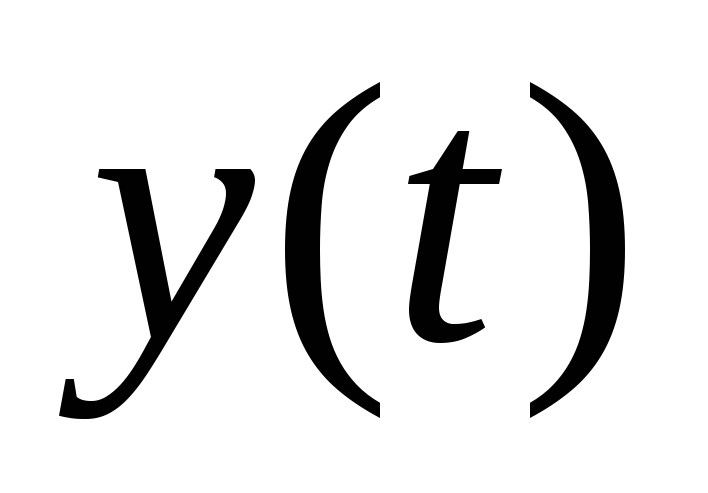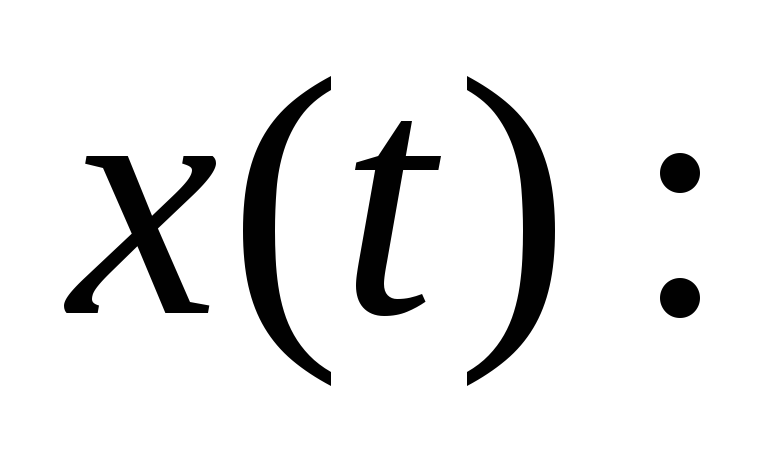# Review of Transfer Function What is Transfer Function? Transfer function is a type of the mathematical models of dynamic systems

 EE 499: Control System Design Identification Identification of Transfer Functions 2.1.1 Review of Transfer Function What is Transfer Function? Transfer function is a type of the mathematical models of dynamic systems.: the output of the dynamic system being studiedthe input of the dynamic system System Model: mathematical description of the dynamic relationship between the input and output of the system. For example, a differential equation can be considered as a mathematical model from the system point of view.Share with your friends: